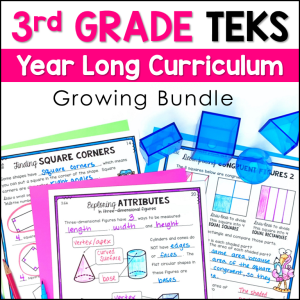Save 10% on your first order with code EXTRA10

# 3rd Grade One & Multi-Step Word Problems

\$4.00\$151.25
SKU: 3794291 Category:

## Description

This set of One-Step and Multi-Step Word Problem Puzzles will engage your students while giving meaningful problem solving and strip diagram practice at Guided Math Stations! The one-step problems all use multiplication or division, while the multi-step problems pair multiplication or division with addition or subtraction.

Classroom Uses:

??Guided Math Stations

??Tutoring

??Small Group Lessons

??Review

??Test Prep

This set includes 8 puzzles that each contain 4 pieces.

4 Puzzle Pieces:

?Word Problem

?Strip Diagram

?Equation(s)

?Bonus Card: Estimation, Arrays, Extra Infromation, etc.

Student Recording Pages are included! There are 2 versions to allow you to choose the best fit for your needs.

?The 1st version is a 1 page condensed version that allows students to write the solution to the problem and the letters of the matching cards.

?The 2nd version is a 4-page full version that gives workspace and plenty of room for students to write out the equation(s) and draw the strip diagram that goes with each problem. This version could actually be used as a stand-alone printable! (Just have students leave the bonus card box blank)

A Complete Teacher Key is included.

There are 2 versions of this resource included inside. I am a firm believer that every resource should be specific to the standards a teacher should teach, and the TEKS and Common Core are definitely not the same! Puzzles 1-4 are exactly the same in each version. Puzzles 5-8 use different numbers in each version.

TEKS Version: Includes some problems that use 2×1 Multiplication (i.e. 48 x 7)

Common Core Version: Includes some problems that use Multiples of 10 x 1-digit Multiplication ( i.e. 40 x 7)

??TEKS Alignment??

3.4(K) solve one-step and two-step problems involving multiplication and division within 100 using strategies based on objects; pictorial models, including arrays, area models, and equal groups; properties of operations; or recall of facts

3.5(B) represent and solve one- and two-step multiplication and division problems within 100 using arrays, strip diagrams, and equations

Supporting Standards

3.4(B) round to the nearest 10 or 100 or use compatible numbers to estimate solutions to addition and subtraction problems

3.4(F) recall facts to multiply up to 10 by 10 with automaticity and recall the corresponding division facts

3.4(G) use strategies and algorithms, including the standard algorithm, to multiply a two-digit number by a one-digit number. Strategies may include mental math, partial products, and the commutative,

associative and distributive properties

3.4(H) determine the number of objects in each groups when a set of objects is partitioned into equal shares or a set of objects is shared equally

3.4(J) determine a quotient using the relationship between multiplication and division

??Common Core Alignment??

3.OA.A.2

Interpret whole-number quotients of whole numbers, e.g., interpret 56 ÷ 8 as

the number of objects in each share when 56 objects are partitioned equally

into 8 shares, or as a number of shares when 56 objects are partitioned into

equal shares of 8 objects each.

3.OA.A.3

Use multiplication and division within 100 to solve word problems in situations

involving equal groups, arrays, and measurement quantities, e.g., by using

drawings and equations with a symbol for the unknown number to represent the

problem.

3.OA.C.7

Fluently multiply and divide within 100, using strategies such as the

relationship between multiplication and division (e.g., knowing that 8 × 5 =

40, one knows 40 ÷ 5 = 8) or properties of operations. By the end of Grade 3,

3.OA.D.8

Solve two-step word problems using the four operations. Represent these problems

using equations with a letter standing for the unknown quantity. Assess the

reasonableness of answers using mental computation and estimation strategies

including rounding.

3.NBT.A.1

Use place value understanding to round whole numbers to the nearest 10 or 100.